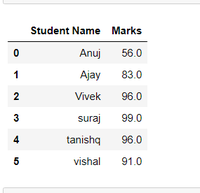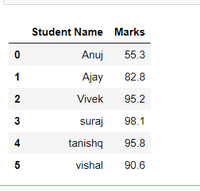GeeksforGeeks App
Open AppBrowser
Continue

# Ceil and floor of the dataframe in Pandas Python – Round up and Truncate

In this article, we will discuss getting the ceil and floor values of the Pandas Dataframe. First, Let’s create a dataframe.

Example:

## Python3

 `# importing pandas and numpy``import` `pandas as pd``import` `numpy as np`  `# Creating a DataFrame``df ``=` `pd.DataFrame({``'Student Name'``: [``'Anuj'``, ``'Ajay'``, ``'Vivek'``,``                                    ``'suraj'``, ``'tanishq'``, ``'vishal'``],``                   ``'Marks'``: [``55.3``, ``82.76``, ``95.23``, ``98.12``,``                             ``95.78``, ``90.56``]})` `df`

Output:### Getting the Ceil Value

We can get the ceil value using the ceil() function. Ceil() is basically used to round up the values specified in it. It rounds up the value to the nearest greater integer.

Example:

## Python3

 `# using np.ceil to round to``# nearest greater integer for``# 'Marks'``df[``'Marks'``] ``=` `df[``'Marks'``].``apply``(np.ceil)` `df`

Output:### Getting the floor value

We can get the floor value using the floor() function. Floor() is basically used to truncate the values. Basically, it truncates the values to their nearest smaller integer.

Example:

## Python3

 `# using np.floor to``# truncate the 'Marks' column``df[``'Marks'``] ``=` `df[``'Marks'``].``apply``(np.floor)` `df`

Output:### Getting the round off value

round() is also used in this purpose only as it rounds off the value according to their decimal digits. Here we can give that to how many decimal places we have to round off our data.

Example:

## Python3

 `# using round() to round up or``# down acc. to their decimal digits``df[``'Marks'``] ``=` `df[``'Marks'``].``round``(decimals``=``1``)` `df`

Output:My Personal Notes arrow_drop_up